# Inventory Management: Is profit maximization right for you?

This entry is part 2 of 4 in the series Predictive Analytics

### Introduction

In the following we will exemplify how sales forecasts can be used to set inventory levels in single or in multilevel warehousing. By inventory we will mean a stock or store of goods; finished goods, raw materials, purchased parts, and retail items. Since the problem discussed is the same for both production and inventory, the two terms will be used interchangeably.

Good inventory management is essential to the successful operation for most organizations both because of the amount of money the inventory represents and the impact that inventories have on the daily operations.

An inventory can have many purposes among them the ability:

1. to support independence of operations,
2. to meet both anticipated and variation in demand,
3. to decouple components of production and allow flexibility in production scheduling and
4. to hedge against price increases, or to take advantage of quantity discounts.

The many advantages of stock keeping must however be weighted against the costs of keeping the inventory. This can best be described as the “too much/too little problem”; order too much and inventory is left over or order too little and sales are lost.

This can be as a single-period (a onetime purchasing decision) or a multi-period problem, involving a single warehouse or multilevel warehousing geographically dispersed. The task can then be to minimize the organizations total cost, maximize the level of customer service, minimize ‘loss’ or maximize profit etc.

Whatever the purpose, the calculation will have to be based on knowledge of the sales distribution. In addition, sales will usually have a seasonal variance creating a balance act between production, logistic and warehousing costs. In the example given below the sales forecasts will have to be viewed as a periodic forecast (month, quarter, etc.).

We have intentionally selected a ‘simple problem’ to highlight the optimization process and the properties of the optimal solution. The last is seldom described in the standard texts.

### The News-vendor problem

The news-vendor is facing a onetime purchasing decision; to maximize expected profit so that the expected loss on the Qth unit equals the expected gain on the Qth unit:

I., where

Co = The cost of ordering one more unit than what would have been ordered if demand had been known – or the increase in profit enjoyed by having ordered one fewer unit,

Cu = The cost of ordering one fewer unit than what would have been ordered if demand had been known  – or the increase in profit enjoyed by having ordered one more unit, and

F(Q) = Demand Probability for q<= Q. By rearranging terms in the above equation we find:

II.This ratio is often called the critical ratio (CR). The usual way of solving this is to assume that the demand is normal distributed giving Q as:

III., where:, is normal distributed with zero mean and variance equal  one.

Demand unfortunately, rarely haves a normal distribution and to make things worse we usually don’t know the exact distribution at all. We can only ‘find’ it by Monte Carlo simulation and thus have to find the Q satisfying the equation (I) by numerical methods.

For the news-vendor the inventory level should be set to maximize profit given the sales distribution. This implies that the cost of lost sales will have to be weighed against the cost of adding more to the stock.

If we for the moment assume that all these costs can be regarded as fixed and independent of the inventory level, then the product markup (% of cost) will determine the optimal inventory level:

IV.In the example given here the critical ratio is approx. 0.8.  The question then is if the inventory levels indicated by that critical ratio always will be the best for the organization.

### Expected demand

The following graph indicates the news-vendors demand distribution. Expected demand is 2096 units1, but the distribution is heavily skewed to the right2  so there is a possibility of demand exceeding the expected demand: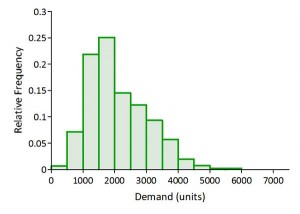By setting the product markup – in the example below it is set to 300% – we can calculate profit and loss based on the demand forecast.

### Profit and Loss (of opportunity)

In the following we have calculated profit and loss as:

Profit = sales less production costs of both sold and unsold items
Loss = value of lost sales (stock-out) and the cost of having produced and stocked more than can be expected to be sold

The figure below indicates what will happen as we change the inventory level. We can see as we successively move to higher levels (from left to right on the x-axis) that expected profit (blue line) will increase to a point of maximum  ¤4963 at a level of 2729 units: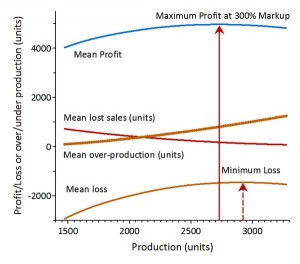At that point we can expect to have some excess stock and in some cases also lost sales. But regardless, it is at this point that expected profit is maximized, so this gives the optimal stock level.

Since we include both costs of sold and unsold items, the point giving expected maximum profit will be below the point minimizing expected loss –¤1460 at a production level of 2910 units.

Given the optimal inventory level (2729 units) we find the actual sales frequency distribution as shown in the graph below. At this level we expect an average sale of 1920 units – ranging from 262 to 2729 units3.

The graph shows that the distribution possesses two different modes4 or two local maxima. This bimodality is created by the fact that the demand distribution is heavily skewed to the right so that demand exceeding 2729 units will imply 2729 units sold with the rest as lost sales.This bimodality will of course be reflected in the distribution of realized profits. Have in mind that the line (blue) giving maximum profit is an average of all realized profits during the Monte Carlo simulation given the demand distribution and the selected inventory level. We can therefore expect realized profit both below and above this average (¤4963) – as shown in the frequency graph below: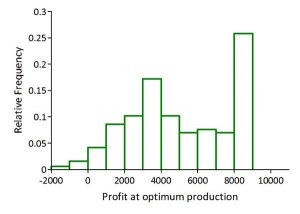Expected (average) profit is ¤4963, with a minimum of ¤1681 and a maximum of ¤8186, the range of realized profits is therefore very large5 ¤9867.

So even if we maximize profit we can expect a large variation in realized profits, there is no way that the original uncertainty in the demand distribution can be reduced or removed.

### Risk and Reward

Increased profit comes at a price: increased risk. The graph below describes the situation; the blue curve shows how expected profit increases with the production or inventory (service) level. The spread between the green and red curves indicates the band where actual profit with 80% probability will fall. As is clear from the graph, this band increases in width as we move to the right indicating an increased upside (area up to the green line) but also an increased probability for a substantial downside (area down to the red line:For some companies – depending on the shape of the demand distribution – other concerns than profit maximization might therefore be of more importance – like predictability of results (profit). The act of setting inventory or production levels should accordingly be viewed as an element for the boards risk assessments.

On the other hand will the uncertainty band around loss as the service level increases decrease. This of course lies in the fact that loss due to lost sales diminishes as the service level increases and the that the high markup easily covers the cost of over-production.Thus a strategy of ‘loss’ minimization will falsely give a sense of ‘risk minimization’, while it in reality increases the uncertainty of future realized profit.

### Product markup

The optimal stock or production level will be a function of the product markup. A high markup will give room for a higher level of unsold items while a low level will necessitate a focus on cost reduction and the acceptance of stock-out: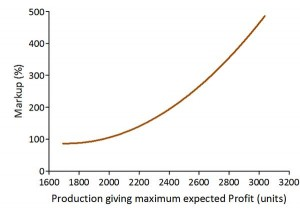The relation between markup (%) and the production level is quadratic6  implying that markup will have to be increasingly higher, the further out on the right tail we fix the production level.

### The Optimal inventory (production) level

If we put it all together we get the chart below. In this the green curve is the accumulated sales giving the probability of the level of sales and the brown curve the optimal stock or production level given the markup.

The optimal stock level is then found by drawing a line from the right markup axis (right y-axis) to the curve (red) for optimal stock level, and down to the x-axis giving the stock level. By continuing the line from the markup axis to the probability axis (left y-axis) we find the probability level for stock-out (1-the cumulative probability) and the probability for having a stock level in excess of demand:By using the sales distribution we can find the optimal stock/production level given the markup and this would not have been possible with single point sales forecasts – that could have ended up almost anywhere on the curve for forecasted sales.

Even if a single point forecast managed to find expected sales – as mean, mode or median – it would have given wrong answers about the optimal stock/production level, since the shape of the sales distribution would have been unknown.

In this case with the sales distribution having a right tail the level would have been to low – or with low markup, to high. With a left skewed sales distribution the result would have been the other way around: The level would have been too high and with low markup probably too low.

In the case of multilevel warehousing, the above analyses have to be performed on all levels and solved as a simultaneous system.

### The state of affairs at the point of maximum

To have the full picture of the state of affairs at the point of maximum we have to take a look at what we can expect of over- and under-production. At the level giving maximum expected profit we will on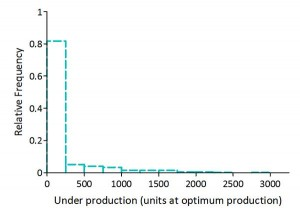average have an underproduction of 168 units, ranging from zero to nearly 30007. On the face of it this could easily be interpreted as having set the level to low, but as we shall see that is not the case.

Since we have a high markup, lost sales will weigh heavily in the profit maximization and as a result of this we can expect to have unsold items in our stock at the end of the period. On average we will have a little over 800 units left in stock, ranging from zero to nearly 2500. The lower quartile is 14 units and the upper is 1300 units so in 75% of the cases we will have an overproduction of less than 1300 units. However in 25% of the cases the overproduction will be in the range from 1300 to 2500 units.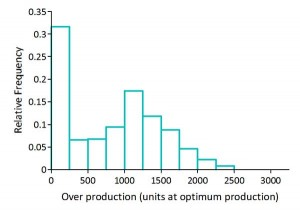Even with the possibility of ending up at the end of the period with a large number of unsold units, the strategy of profit maximization will on average give the highest profit. However, as we have seen, with a very high level of uncertainty about the actual profit being realized.

Now, since a lower inventory level in this case only will reduce profit by a small amount but lower the confidence limit by a substantial amount, other strategies giving more predictability for the actual result should be considered.

Series Navigation<< Forecasting sales and forecasting uncertaintyInventory management – Some effects of risk pooling >>
1. Median demand is 1819 units and the demand lies most typically in the range of 1500 to 2000 units []
2. The demand distribution has a skewness of 0.78., with a coefficient of variation of 0.45, a lower quartile of 1432 units and an upper quartile of 2720 units. []
3. Having a lower quartile of 1430 units and an upper quartile of 2714 units. []
4. The most common value in a set of observations. []
5. Having a lower quartile of ¤2991 and an upper quartile of ¤8129. []
6. Markup (%) = 757.5 – 0.78*production level + 0.00023*production level2 []
7. Having a coefficient of variation of almost 250% []